Electric Fields and Forces AP Physics B Electric

• Slides: 17Electric Fields and Forces AP Physics B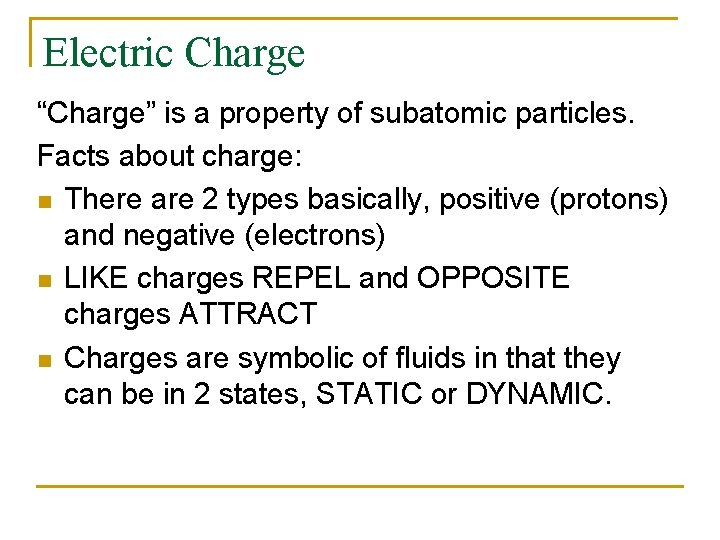Electric Charge “Charge” is a property of subatomic particles. Facts about charge: n There are 2 types basically, positive (protons) and negative (electrons) n LIKE charges REPEL and OPPOSITE charges ATTRACT n Charges are symbolic of fluids in that they can be in 2 states, STATIC or DYNAMIC.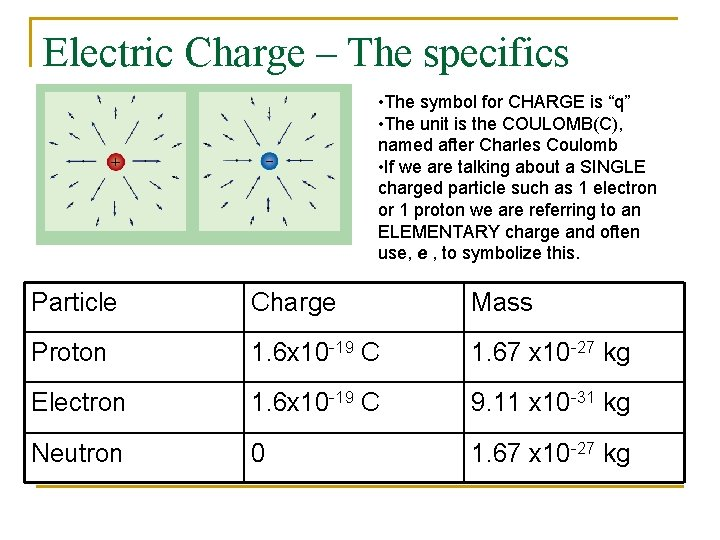Electric Charge – The specifics • The symbol for CHARGE is “q” • The unit is the COULOMB(C), named after Charles Coulomb • If we are talking about a SINGLE charged particle such as 1 electron or 1 proton we are referring to an ELEMENTARY charge and often use, e , to symbolize this. Some important constants: Particle Charge Mass Proton 1. 6 x 10 -19 C 1. 67 x 10 -27 kg Electron 1. 6 x 10 -19 C 9. 11 x 10 -31 kg Neutron 0 1. 67 x 10 -27 kgCharge is “CONSERVED” Charge cannot be created or destroyed only transferred from one object to another. Even though these 2 charges attract initially, they repel after touching. Notice the NET charge stays the same.Conductors and Insulators The movement of charge is limited by the substance the charge is trying to pass through. There are generally 2 types of substances. Conductors: Allow charge to move readily though it. Insulators: Restrict the movement of the charge Conductor = Copper Wire Insulator = Plastic sheath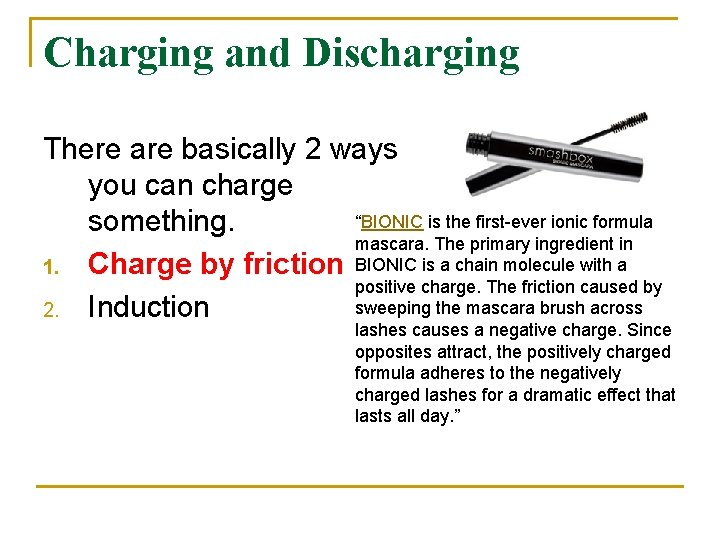Charging and Discharging There are basically 2 ways you can charge “BIONIC is the first-ever ionic formula something. mascara. The primary ingredient in 1. Charge by friction BIONIC is a chain molecule with a positive charge. The friction caused by sweeping the mascara brush across 2. Induction lashes causes a negative charge. Since opposites attract, the positively charged formula adheres to the negatively charged lashes for a dramatic effect that lasts all day. ”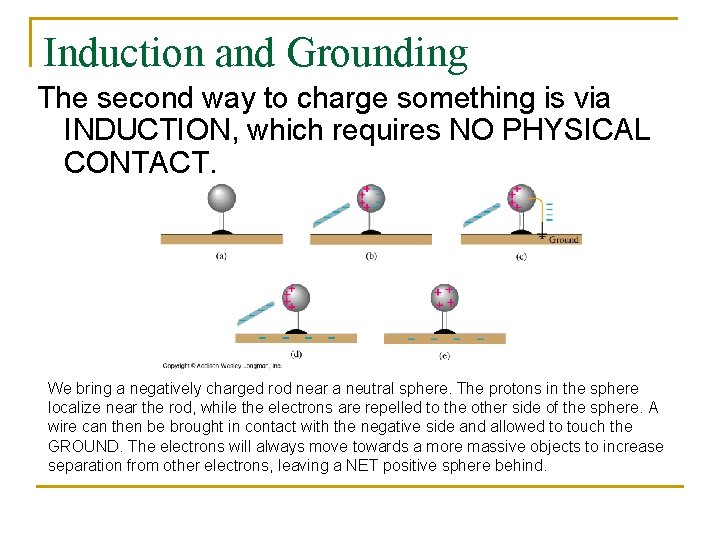Induction and Grounding The second way to charge something is via INDUCTION, which requires NO PHYSICAL CONTACT. We bring a negatively charged rod near a neutral sphere. The protons in the sphere localize near the rod, while the electrons are repelled to the other side of the sphere. A wire can then be brought in contact with the negative side and allowed to touch the GROUND. The electrons will always move towards a more massive objects to increase separation from other electrons, leaving a NET positive sphere behind.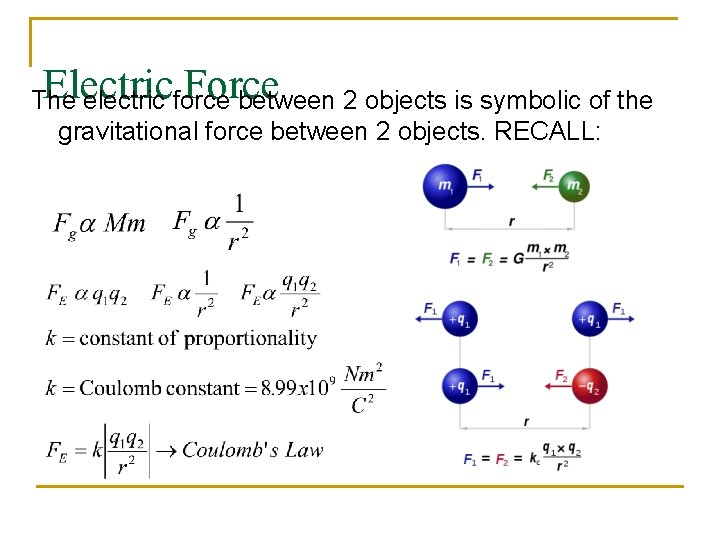Electric Force The electric force between 2 objects is symbolic of the gravitational force between 2 objects. RECALL:Electric Forces and Newton’s Laws Electric Forces and Fields obey Newton’s Laws. Example: An electron is released above the surface of the Earth. A second electron directly below it exerts an electrostatic force on the first electron just great enough to cancel out the gravitational force on it. How far below the first electron is the second? Fe e mg e r=? 5. 1 m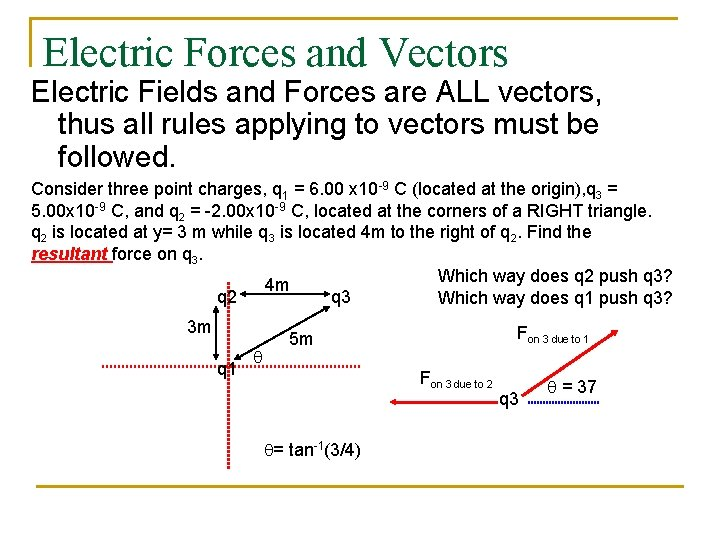Electric Forces and Vectors Electric Fields and Forces are ALL vectors, thus all rules applying to vectors must be followed. Consider three point charges, q 1 = 6. 00 x 10 -9 C (located at the origin), q 3 = 5. 00 x 10 -9 C, and q 2 = -2. 00 x 10 -9 C, located at the corners of a RIGHT triangle. q 2 is located at y= 3 m while q 3 is located 4 m to the right of q 2. Find the resultant force on q 3. Which way does q 2 push q 3? 4 m q 2 q 3 Which way does q 1 push q 3? 3 m q 1 q Fon 3 due to 1 5 m Fon 3 due to 2 q= tan-1(3/4) q 3 q = 374 m Exampleq 2 Cont’ q 3 3 m q 1 q Fon 3 due to 1 5 m q= tan-1(3/4) Fon 3 due to 2 q 3 q = 37 F 3, 1 sin 37 F 3, 1 cos 37 5. 6 x 10 -9 N 7. 34 x 10 -9 N 1. 1 x 10 -8 N 64. 3 degrees above the +x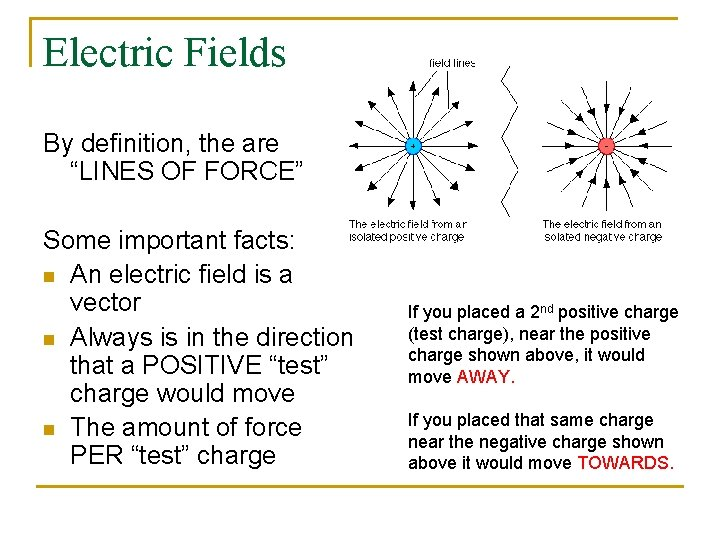Electric Fields By definition, the are “LINES OF FORCE” Some important facts: n An electric field is a vector n Always is in the direction that a POSITIVE “test” charge would move n The amount of force PER “test” charge If you placed a 2 nd positive charge (test charge), near the positive charge shown above, it would move AWAY. If you placed that same charge near the negative charge shown above it would move TOWARDS.Electric Fields and Newton’s Laws Once again, the equation for ELECTRIC FIELD is symbolic of the equation for WEIGHT just like coulomb’s law is symbolic of Newton’s Law of Gravitation. The symbol for Electric Field is, “E”. And since it is defined as a force per unit charge he unit is Newtons per Coulomb, N/C. NOTE: the equations above will ONLY help you determine the MAGNITUDE of the field or force. Conceptual understanding will help you determine the direction. The “q” in the equation is that of a “test charge”.Example An electron and proton are each placed at rest in an external field of 520 N/C. Calculate the speed of each particle after 48 ns What do we know me=9. 11 x 10 -31 kg 8. 32 x 10 -19 N mp= 1. 67 x 10 -27 kg qboth=1. 6 x 10 -19 9. 13 x 1013 m/s/s C 4. 98 x 1010 m/s/s vo = 0 m/s E = 520 N/C 4. 38 x 106 m/s t = 48 x 10 -9 s 2. 39 x 103 m/sAn Electric Point Charge As we have discussed, all charges exert forces on other charges due to a field around them. Suppose we want to know how strong the field is at a specific point in space near this charge the calculate the effects this charge will have on other charges should they be placed at that point. POINT CHARGE TEST CHARGE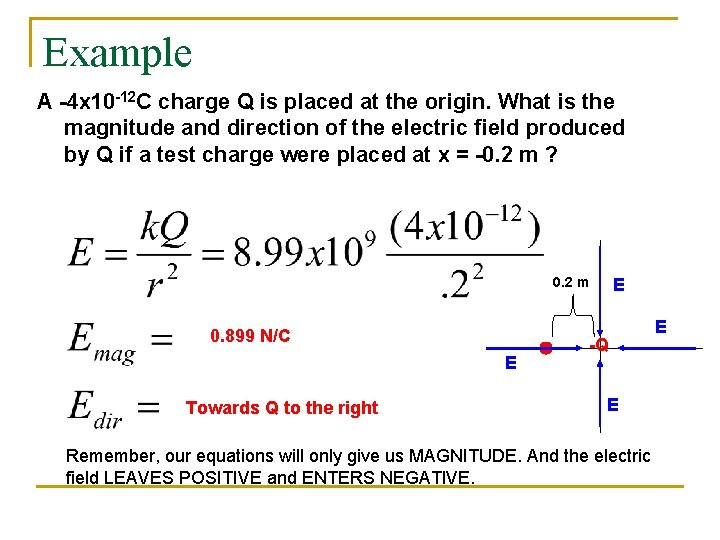Example A -4 x 10 -12 C charge Q is placed at the origin. What is the magnitude and direction of the electric field produced by Q if a test charge were placed at x = -0. 2 m ? 0. 2 m 0. 899 N/C E Towards Q to the right E -Q E Remember, our equations will only give us MAGNITUDE. And the electric field LEAVES POSITIVE and ENTERS NEGATIVE. E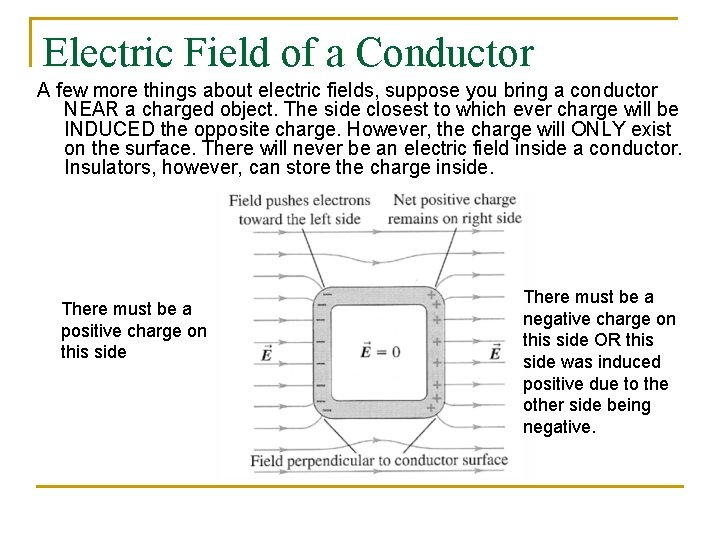Electric Field of a Conductor A few more things about electric fields, suppose you bring a conductor NEAR a charged object. The side closest to which ever charge will be INDUCED the opposite charge. However, the charge will ONLY exist on the surface. There will never be an electric field inside a conductor. Insulators, however, can store the charge inside. There must be a positive charge on this side There must be a negative charge on this side OR this side was induced positive due to the other side being negative.